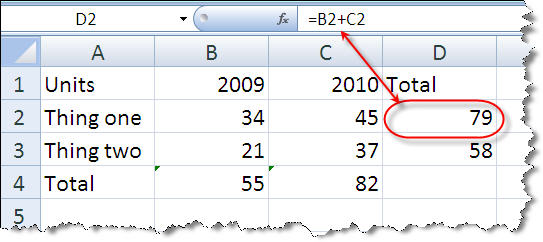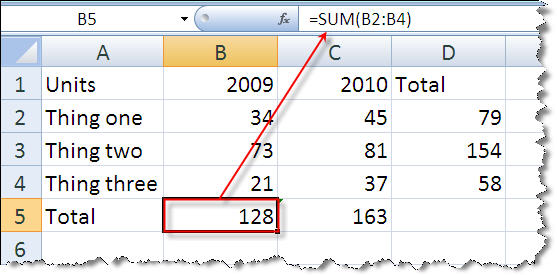You probably know this already, but I’ll go over it just in case. I recommend using Business Plan Pro software so you don’t have to do this, but it’s good to know anyhow, and you can certainly do everything in this book without that software. So here’s a bit about spreadsheets.

Spreadsheets are normally arranged in rows and columns, with rows numbered from 1 to whatever, and columns labeled from A to whatever. Simple mathematical formulas refer to the cells that are identified by row and column. For example:So what we see here is a simple formula that adds the 34 in cell B2 to the 45 in cell C2 to get the sum of those two, which is 79. That number is in cell D2, so you see the formula showing at the top when you click on D2. Also the number in the upper left corner indicates which cell the displayed formula belongs to.

Here’s another simple example:In this case the cell named B5 is highlighted, and its formula says to sum up all the cells from B2 to B4. That’s three cells, and the numbers they contain sum up to 128.

There are lots of books and websites and different instructions and tutorials available for spreadsheets. This is enough for now, so you can understand my simple forecast examples.